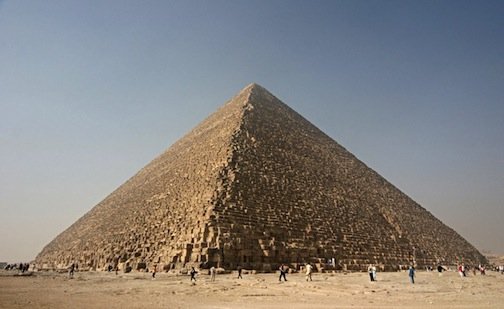The Great Pyramid of Giza

Geometry Level 3The base length of the Pyramid of Giza is $230\text{m}$, the height is $147\text{m}$ and the Pyramid is made of crude limestone which has a density of about $2300\text{kgm}^{-3}$. The Pyramid of Giza is a square pyramid. The mass of the pyramid may be written in scientific notation to three significant figures in the form $\overline{abc} \times 10^{7} \text{kg}$.

What is $\overline{abc}$?

You can compare the mass you calculated with that of the mass listed in the Wikipedia page for the Great Pyramid of Giza. It is in the first paragraph of 'History and Description'.

×